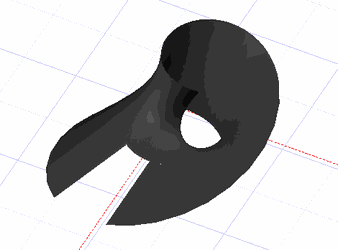# Surface with Law

(Available only in Platinum)

Default UI Menu: Draw/3D Object/3D Law Objects/Surface with Law Ribbon UI Menu: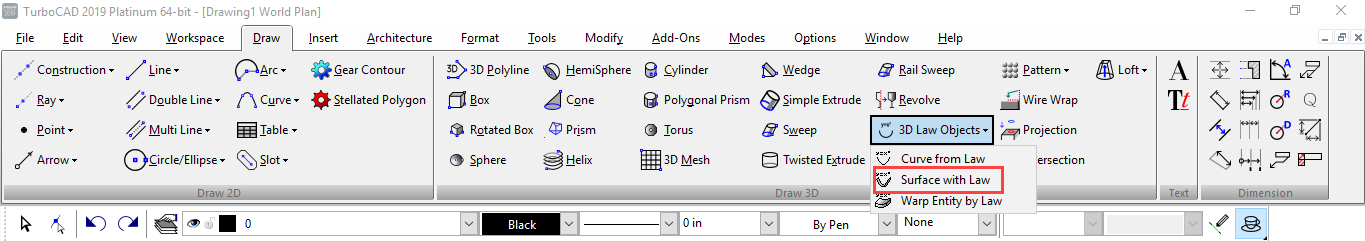This tool creates an open surface using Laws. Laws are mathematical functions in the form of a formula. They are used to define how resulting objects are modified. They are composed and selected in the Laws Editor which is specific to each tool that uses laws and can be opened from that tool.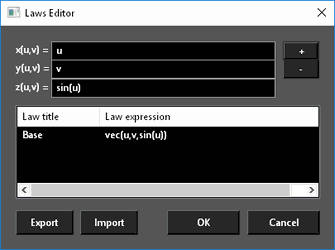To use a the Surface from Laws tool:

1. Select the tool. The Laws Editor opens.
2. Select the desired law in the Laws table by double-clicking and press OK. The law that is applied is what is in the formula field.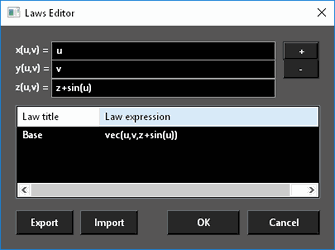3. Set the U-min, U-max and V-min, V-max either by entering a values in the Inspector Bar or by selecting two points. (U-min and V-min default to zero (0))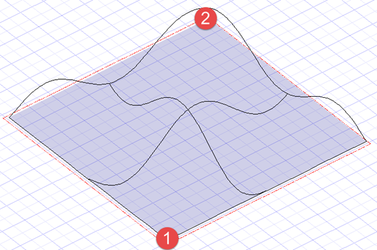To create a law:

1. Type the desired formulas into the formula fields.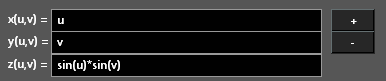1. Press the + (plus) button.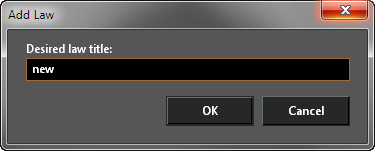1. Type in a titke for the law and press OK. The new law will appear in the Laws table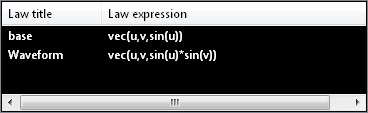To Delete a law:

1. Open the Laws Editor.

2. Click on the Law in the Laws table to select it.

3. Right click and select Delete, or press the - (minus) button.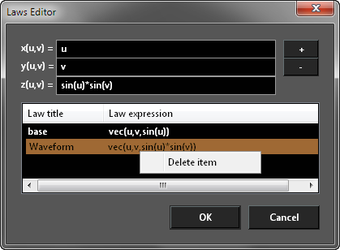Laws cannot be edited but double click a law will load it into the Formula fields where it can be altered and then a new altered law added.

Editing a Surface by Law

The values for a Surface by law can be edited via the Selection Info palette or by the object properties.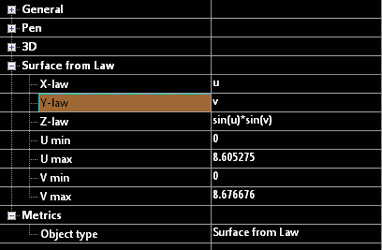To Delete a law:

1. Open the Laws Editor.

2. Click on the Law in the Laws table to select it.

3. Right click and select Delete, or press the - (minus) button.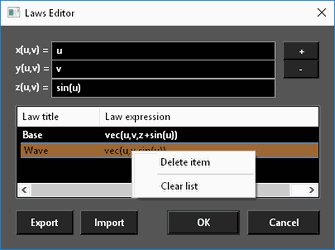Laws cannot be edited but double click a law will load it into the Formula fields where it can be altered and then a new altered law added.

To Delete all laws:

1. Open the Laws Editor.

2. Click on the Law in the Laws table to select it.

3. Right click and select Clear List.To Export laws:

1. Open the Laws Editor.
2. Click Export.
3. Type a file name and click Save.To Import laws:

1. Open the Laws Editor.
2. Click Import.
3. Select a file and click Open.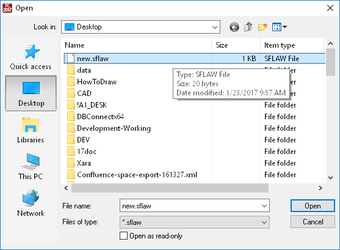x(u,v) y(u,v) z(u,v)

In the Offset tool the functions are defined as a function of U and V. Where U is the distance along the x-axis of the object and V is the distance along the y-axis of the object. Setting x(u,v)=u, y(u,v)=v, z(u,v)=sin(u)sin(v), U min = 0, U max = 9, V min = 0, V max = 9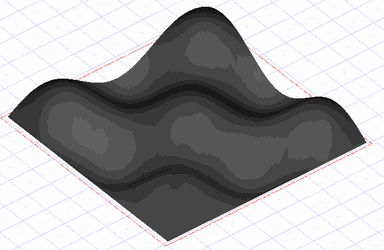Setting x(u,v)=u, y(u,v)=v, z(u,v)=sin(u)cos(v), U min = 0, U max = 9, V min = 0, V max = 9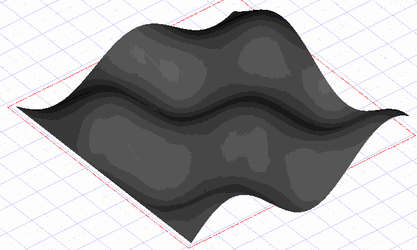Setting: x(u)=2(1-exp(u/(6pi)))cos(u)cos(v/2)^2 y(u)=2(-1+exp(u/(6pi)))sin(u)cos(v/2)^2 z(u)=1-exp(u/(3pi))-sin(v)+exp(u/(6pi))sin(v) U min = 0, U max = 20, V min = 0, V max = 6.283185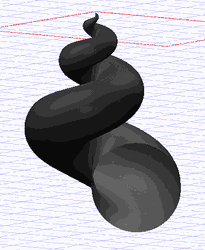Setting: x(u)=(1+v/2cos(u/2))cos(u) y(u)=(1+v/2cos(u/2))sin(u) z(u)=v/2sin(u/2) U min = 0, U max = 12, V min = 0, V max = 3